# Reflection

In Geometry, reflection is one of the four types of transformations. The four basic transformations are

• Translation
• Reflection
• Rotation
• Dilation or Resizing

In this article, let’s discuss the meaning of Reflection in Maths, reflections in the coordinate plane and examples in detail.

## Reflection Definition

In Geometry, a reflection is known as a flip. A reflection is a mirror image of the shape. An image will reflect through a line, known as the line of reflection. A figure is said to reflect the other figure, and then every point in a figure is equidistant from each corresponding point in another figure. The reflected image should have the same shape and size, but the image faces in the opposite direction. In reflection, translation may also occur because of changes in the position. Here, the original image is called pre-image, and its reflection is called the image. The representation of pre-image and image are ABC and A’B’C’, respectively. The reflection transformation may be in reference to the coordinate system (X and Y-axis).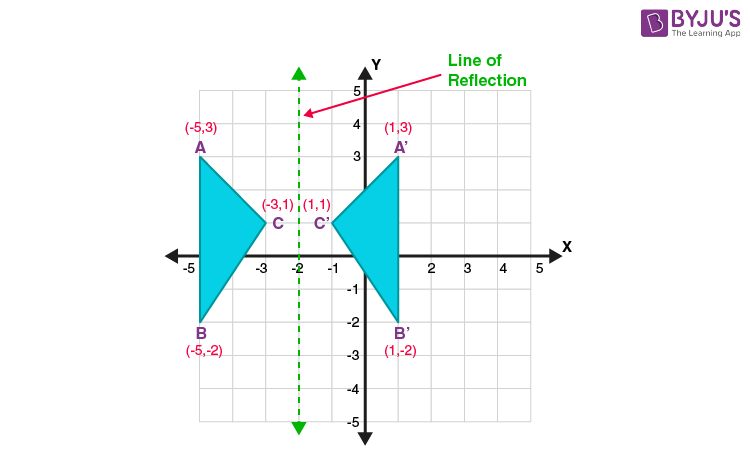## Reflections in the Coordinate Plane

The reflection transformation may be in reference to X and Y-axis.

### Reflection over X-axis

When a point is reflected across the X-axis, the x-coordinates remain the same. But the Y-coordinates are transformed into their opposite signs.

Therefore, the reflection of the point (x, y) across X-axis is (x, -y).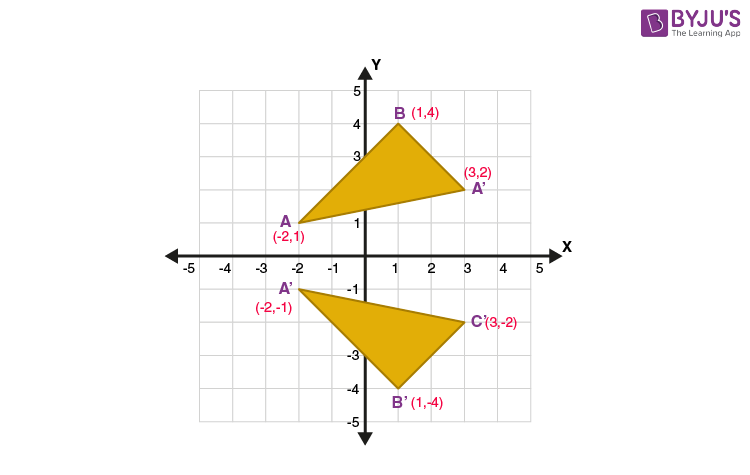### Reflection over Y-axis

When a point is reflected across the Y-axis, the Y-coordinates remain the same. But the X-coordinates is transformed into its opposite signs.

Therefore, the reflection of the point (x, y) across Y-axis is (-x, y).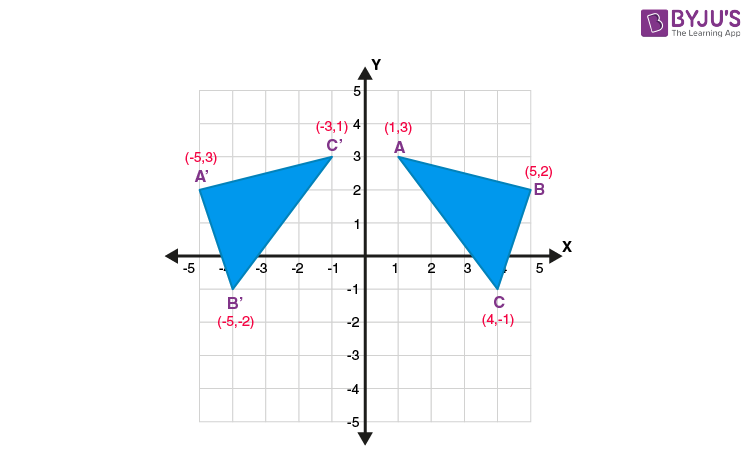### Reflection over Y = X

When a point is reflected across the line y = x, the x-coordinates and y-coordinates change their place. Similarly, when a point is reflected across the line y = -x, the x-coordinates and y-coordinates change their place and are negated.

Therefore,

The reflection of the point (x, y) across the line y = x is (y, x).

The reflection of the point (x, y) across the line y = – x is (-y, -x).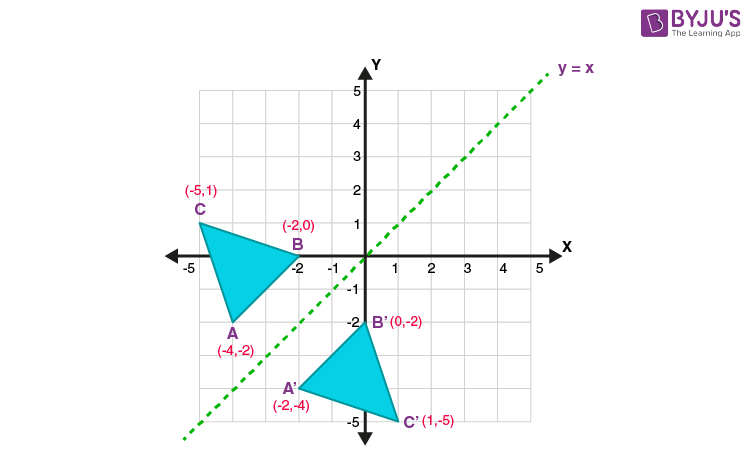## Reflection on a Point

A reflection point occurs when a figure is constructed around a single point known as the point of reflection or centre of the figure. For every point in the figure, another point is found directly opposite to it on the other side. Under the point of reflection, the figure does not change its size and shape.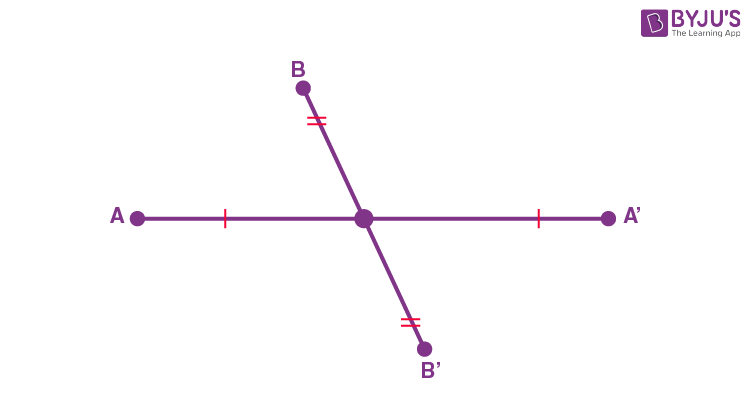### Reflection at origin (0, 0)

In the coordinate plane, we can use any point as the point of reflection. The most commonly used point is “origin”.

Example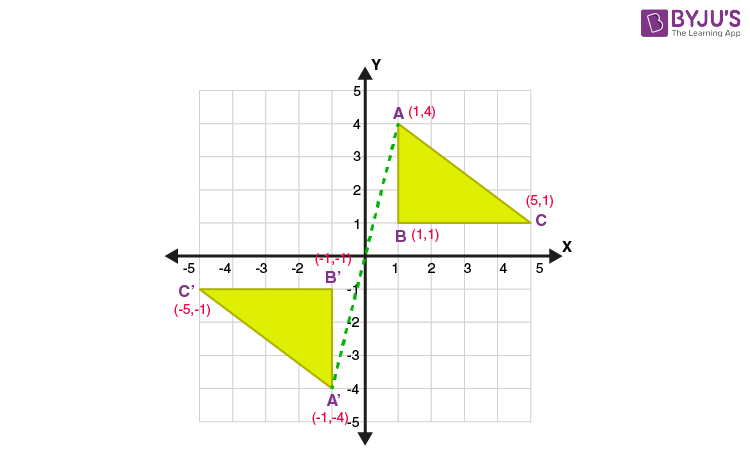Let ABC be the triangle, and the coordinates are A(1,4), B(1,1), and C(5,1). After the point of reflection in origin, the pre-image ABC is transformed into A’B’C’. When you draw a line segment connecting the points A and A’, the origin should be the midpoint of the line.

Therefore,

The point of reflection in origin (0, 0), the image of the point (x, y) is (-x, -y).

Hence, the coordinates of the triangle A’B’C are A’(-1,-4), B’(-1,-1), and C’(-5,-1).

Stay tuned with BYJU’S – The Learning App and explore interesting videos.

 Related Links Transformations Rotation Geometry Coordinate Geometry